# Fermat's last theorem

## History

### Statement of the conjecture

The statement was made by Fermat in the margin of a notebook, with the assertion that he had a beautiful proof that was too long for the margin. However, Fermat never mentioned the result in communications with other authors, and it is possible that he discovered an error in his proof.

When Fermat's notebooks were discovered after his death, the result came to be known as Fermat's last theorem, since Fermat had claimed to have a proof.

### Proof of the conjecture

Further information: History of Fermat's last theorem

The final proof of Fermat's last theorem was provided by Andrew Wiles. He essentially proved a special version of the Taniyama-Shimura-Weil conjecture, building on an observation of Ribet and Frey that linked the conjecture to Fermat's last theorem. The general case of the Taniyama-Shimura-Weil conjecture has also been settled.

## Statement

### Statement in terms of exponential Diophantine

Fermat's last theorem, more aptly termed Fermat's conjecture or the Fermat-Wiles theorem, states the following equivalent things about the equation: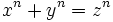.

1. The equation has no solutions forpositive integers anda positive integer greater than.
2. The equation has no solutions forintegers,, anda positive integer greater than.
3. The equation has no solutions forintegers,, andequal to four or equal to a prime greater than.
4. The equation has no solution forintegers,, withpairwise relatively prime, andequal to four or to a prime greater than.

### Statement in terms of rational points on a curve

For, consider the following algebraic curve over the field of rational numbers: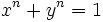.

Then, Fermat's last theorem says the following equivalent things:

1. For all, the only rational points on this curve are the points,, and, ifis even, thenand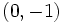.
2. The above holds forand for all prime values of.

### Equivalence of definitions

The equivalence of the definitions involving Diophantine equations is fairly straightforward:

• Definitions (1) and (2) are equivalent because any solution in integers can be rearranged to get a solution with positive integers.
• Definitions (2) and (3) are equivalent because every natural numberis a multiple either ofor of a prime greater than. Thus, anypower is a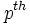power for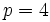ora prime greater than. In particular, finding a counterexample to the theorem for anyis equivalent to finding a counterexample foror a prime greater than.
• Definitions (3) and (4) are equivalent because we can factor out the gcd of, and note that if any two of them have a common factor, the third one shares the common factor.

The equivalence with definitions involving rationals follows from the fact that: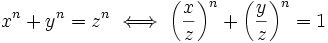.

## Related facts

### Stronger facts and conjectures

• Beal conjecture: This states that the equation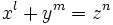has no solution for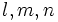all greater thanandpairwise relatively prime.
• Taniyama-Shimura-Weil conjecture
• Euler's false attempted generalization of Fermat's last theorem: Euler attempted to generalize Fermat's last theorem to the statement that nopower can be expressed as a sum of fewer thannonzeropowers. However, this is false, since there are examples of fourth powers expressible as a sum of three fourth powers.
• abc conjecture implies Fermat's last theorem for sufficiently large exponents.

### Analogous facts over other rings

• Fermat's last theorem for polynomial rings over fields of characteristic zero: The analogue of Fermat's last theorem holds for polynomial rings over fields of characteristic zero: the equationhas no solution for polynomialswhere not all polynomials are constant polynomials, and none of them is zero.

### The case of squares

Further information: Classification of primitive Pythagorean triples

There are infinitely many solutions to the equation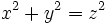, and the primitive solutions (i.e., the solutions with a gcd of) correspond to the rational points on the circle. Each primitive solution is termed a Pythagorean triple.

Waring's problem asks whether, for every natural number, there exists a number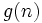such that every natural number can be expressed as a sum of at mostpowers. The problem has an affirmative answer, though there is no known formula to computefor every natural number.

## Particular cases

### The caseFurther information: Fermat's last theorem for fourth powers

Fermat's last theorem for fourth powers can be proved by a method of infinite descent, using the classification of primitive Pythagorean triples, i.e., of solutions to. In fact, the following stronger version can be shown: no square can be expressed as a difference of nonzero fourth powers.

### The caseand regular primes

Further information: Fermat's last theorem for cubes, Fermat's last theorem for regular primes

Fermat's last theorem for cubes is easy to prove. The method generalizes to proving Fermat's last theorem for any regular prime. A regular prime is a prime for which the corresponding cyclotomic ring of integers (the ring of integers obtained by adjoining a primitiveroot of unity) is a unique factorization domain. In this case, the expression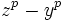can be factorized using theroots, and the unique factorization domain property can be used effectively.

The smallest irregular prime is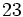. The infinitude of irregular primes is known, though the infinitude of regular primes is still a conjecture. Thus, this approach does not even show Fermat's last theorem to be true for infinitely many exponents.